# Euler's Equation

## Euler's equation

[′ȯi·lərz i¦kwā·zhən]
(mathematics)

## Euler’s Equation

(1) A differential equation of the form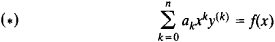where a0, . . ., an are constants. When x > 0, equation (*) reduces, by the substitution x = et, to a linear differential equation with constant coefficients. Equation (*) was studied by L. Euler in 1740. The equation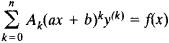reduces to it through the substitution x’ = ax + b.

(2) A differential equation of the form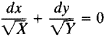where X(x) = a0x4 + a1x3 + a2x2 + a3x + a4 and Y(y) = a0y4 + a1y3 + a2y2 + a3y + a4. Euler considered this equation in a number of works, the first of which dates from 1753. He showed that the general solution of the equation is a symmetric polynomial of degree 4 in x and y. This result provided the basis for the theory of elliptic integrals.

(3) A differential equation of the form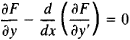which is used in the calculus of variations to search for extrema of the integral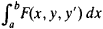The equation was derived by L. Euler in 1744.

References in periodicals archive ?
Popa, "Hyers-Ulam stability of Euler's equation," Applied Mathematics Letters, vol.
Many of these entities have been given simple and ambiguous names such as Euler's function, Euler's equation, and Euler's formula.
The latter requires application of Euler's equation from the calculus of variations.
When L is [greater than or equal to] 400 mm, [[sigma].sub.b] obtained by Southwell's method and Engesser's tangent modulus are close to that predicted by Euler's equation. For small L, however, [[sigma].sub.b] obtained by Southwell's method is smaller than that predicted by Euler's equation.
The load carrying capacity of a regular circular column is given by Euler's equation:
The simplest estimate, using Euler's equation for the buckling force on a beam (8), the bending stiffness of a single microtubule decorated with associated proteins [~5 x [10.sup.-23] [Nm.sup.2]] (9), the average number of microtubules of a newt lung kinetochore [~20] (5), and the average measured length of the kinetochore fiber [~10 [mu]], predicts that the buckling force on a 10-[mu]-long and growing kinetochore fiber would be 100 pN!
He converted the problem into an equivalent mathematical form that enabled him to pick out candidates likely to satisfy Euler's equation. A few other mathematicians had tried a similar approach, says Elkies, but either they gave up too soon or they were thinking in terms of proving rather than disproving the conjecture.
Here, the authors complete the proof of the envelope theorem for the class of variational problems concerned, using the corresponding Euler's equation.
He then moves to applications in Fourier series, including strings and springs, and devotes the rest of the text to the calculus of variations, including Euler's Equation, harmonic functions, Hamilton's Action and Lagranges' Equation, finishing with nonEuclidean geometry and some fun with relativity.
Shape optimization of fibers in a composite structure presented in this paper is based on an original formulation of optimal shape problems leading to Euler's equations belonging to the optimization.
The classes of offshore structures are described in a short introduction, followed by a review of fundamental equations and concepts of motion, rotational and irrotational flows, velocity potential, Euler's equations, steam function and other concepts.
Week 6: Euler's equations, the rotator, the symmetric free top, Feynman's plate experiment, geometry of three-dimensional reconstruction of plate from image

Site: Follow: Share:
Open / Close